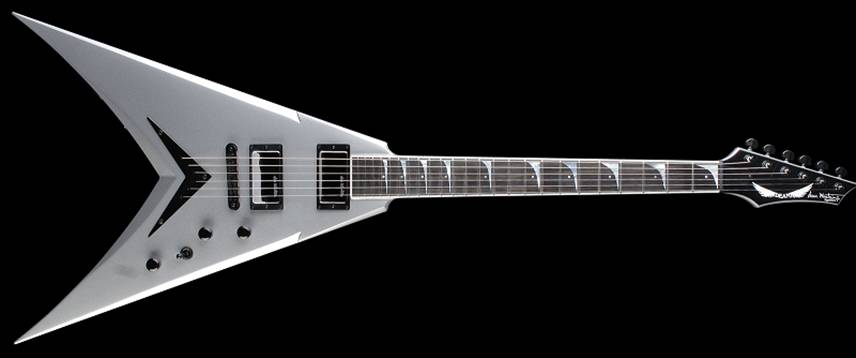“THE MECHANIX” guitar Tuner

Fan(Alex) Wang (FW45)

Ayan Nandi (AN94)

Paul Liew (PCL25)

Introduction: Motivation for Design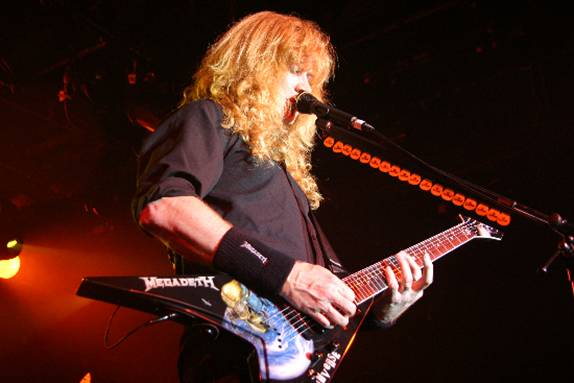The Mechanix is a motorized guitar tuner for a standard 6-string electric or acoustic fixed bridge guitar.  Named in honor of Megadeth guitar legend Dave Mustaine, the Mechanix is a unique and innovative product which has numerous patent possibilities.  Traditional handheld guitar tuners are passive; they tell the user whether the guitar string is in tune, sharp, or flat, but the actual adjustment of the tension in the string has to be done by the user. This requires a fair amount of dexterity from the user, particularly when fine tuning is required. Thus, to increase both the convenience and the precision of the tuning process, we designed an active, motorized guitar tuner. The Mechanix responds to the user plucking one of the strings by turning the tuning knob until the string has reached its Standard Tuning note.

High Level Design

Rationale and sources of project idea

Passive guitar tuners have been commercially available for a long time and have also been implemented as ECE476 projects before, but none have incorporated a machine-controlled tuning process. The Mechanix is aimed at automating the guitar tuning process as much as possible, so as to make the process of tuning more precise and more convenient.

Background math

Standard Tuning, the most popular tuning on a 6-string guitar, is as follows:

 String # Note Frequency (Hz) 1 (Highest) E 329.6 2 B 246.9 3 G 196.0 4 D 146.8 5 A 110.0 6 (Lowest) E 82.4

Tuning the guitar involves adjusting the tension in each string until it vibrates at its Standard Tuning frequency.

Logical Structure

Our project can be divided into two main stages: signal processing and driving the motor.

Signal Processing

The signal from the plucked string, having a peak-to-peak amplitude on the order of tens of millivolts, first went through a preamplifier that scaled the amplitude up to the order of one volt. It was then put through a bandlimiting filter—a hardware low-pass filter with a cutoff of 400 Hz—before being sampled by the A/D converter on the microcontroller, so that the sampled signal would not be distorted by aliasing. The sampled signal was then filtered in software using Butterworth filters to suppress the higher harmonics of the vibrating string; the aim of this was to yield, as far as possible, a clean sine wave of the fundamental frequency of the plucked string. The frequency of this filtered signal was then calculated by the method of counting zero crossings—noting the time taken for the sine wave to rise through a threshold voltage 20 times, and dividing this time by 20 to get the period of the wave, and taking the reciprocal of the period to get the frequency. An LCD display shows the frequency of the vibrating string.

Driving the motor

To connect the rotating shaft of the motor to a tuning knob of the guitar, we needed a ‘claw’ fixed to the motor that would grasp the tuning knob. We had the claw made in the machine shop of the Department of Mechanical and Aerospace Engineering (special thanks to M&AE ’09 students Patxi Fernandez-Zelaia and Adeboye Ajayi for machining the part). We also estimated the torque required to turn the tuning knobs and, with some assistance and direction from Professor Francis Moon, we obtained a stepper motor from Rick Schmidt of the M&AE department that produced the torque required to turn the tuning knobs and met our design needs.

The motor was driven based on the frequency calculated in the Signal Processing stage. If the frequency calculated was within 1% of the Standard Tuning frequency of the string, the motor was halted. If the frequency calculated exceeded the Standard Tuning frequency by more than 1%, a signal was sent to the motor to rotate the tuning knob in the direction that would loosen the string. If the frequency calculated was below the Standard Tuning by more than 1%, a signal was sent to instruct the motor to rotate in the string-tightening direction. Because we used a stepper motor, we were able to control the turning of the tuning knobs with great precision (to the nearest 1.8o, which was the step size of the motor).

The motor took as inputs PWM signals that would have required a rather complicated implementation if done in software. We avoided this by using instead a motor driver chip, which interfaced between the microcontroller and the motor and which produced the required PWM signals to drive the motor based on simple logical signals (0 or 1) from the microcontroller. This made our motor-driving code clean and simple.

Relationship of our design to available IEEE, ISO, ANSI, DIN, and other standards

We programmed the microcontroller in ANSI C. We used the IEEE 32-bit floating point for the floating point calculations required in calculating frequencies and filter coefficients.

Program/Hardware Design: Signal Processing Implementation

We now aim to provide a cursory overview of the entire process of calculating the frequency of a plucked guitar string, and consequently elaborate each specific piece in copious detail.  The Signal Processing design uses both analog hardware as well as software methods to output a frequency for a plucked guitar string signal.

Below is a simple flowchart detailing the entire process: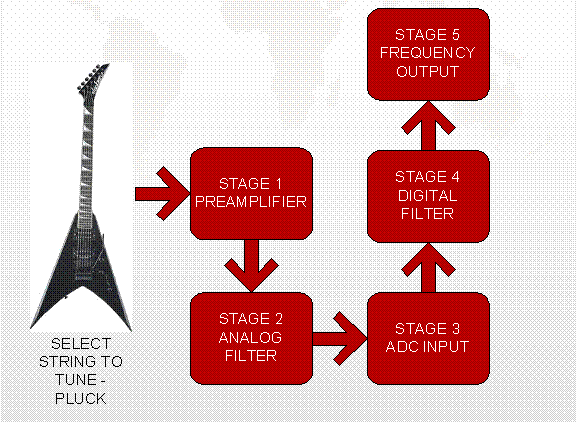STAGE 1 - PREAMPLIFIER

The line output from the guitar is weak and has a low current.  In order to be able to actually read this signal, we would need to use a BJT amplifier.  To circumvent this cumbersome task, we used an Audio Buddy Preamplifier I already had at home.  This gave this signal a boost and removed some noise as well.

STAGE 2 – ANALOG FILTER

The analog signal output from the preamp is of infinite bandwidth and contains a large amount of second and third harmonic.  It also has a random DC bias.  Before the signal can be send to the microcontroller ADC, it must be band-limited to remove aliasing.  The biasing circuit first uses a 1 uF capacitor to remove any DC bias the signal may have.  We then used a voltage divider to bias the signal at 2.5 V, the middle of the MCU conversion range.  We then passed it through a Salen Key 2nd order low pass Butterworth to band limit the signal to 400 Hz.    Below is a detailed diagram of the biasing circuit and the analog filter:

The analog filter uses a cutoff of 400 Hz, and the resistances and capacitances were chosen by the equations below: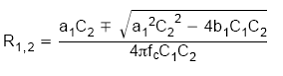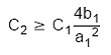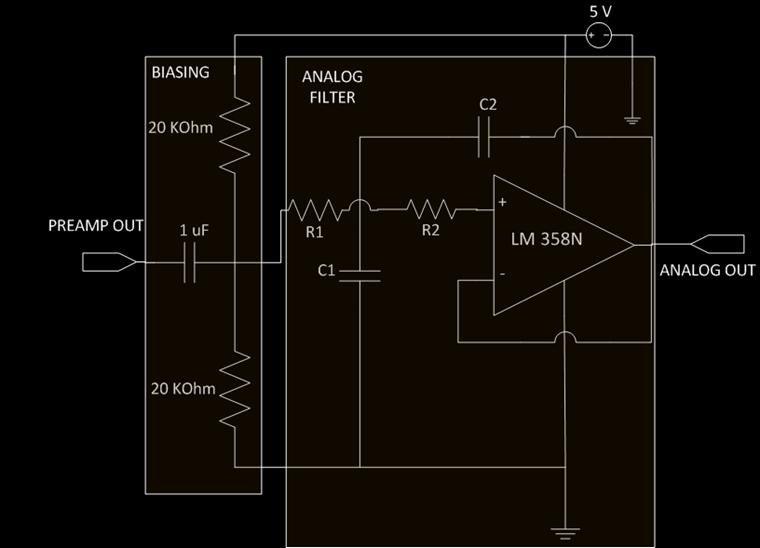The filter has transfer function:

A(s) = 1/(1 + 1.4142s + s^2)

STAGE 3 – ADC INPUT

The output of Stage 2 is sent to the ADC0 input of the ATMEGA32 and a reference voltage of 5 V is used for sampling and quantization.  The sampling rate is 2 kHz, which satisfies the Nyquist requirements for sampling and recovery.  A constant value of 127 was subtracted from the converted value so that the input to the digital filter would be biased at zero and not at 2.5 V.

STAGE 4 – DIGITAL FILTER

We used Bruce Land’s code for a second order filter and modified to accommodate for the added coefficients.  The filter function IIR2() will accommodate for the following transfer function:

Y[n] = b1*X{n] + b2*X[n-1] + b3*X[n-2] – a2*Y[n-1] –a3*Y[n-2]

The converted and zero biased fixed point signal was sent to the filter, which would remove the second harmonic of the guitar signal.  Each string had its own separate filter, which would have the first harmonic in the middle of its band to minimize attenuation.  The filters were able to reject second harmonic at about 8+ dB for the lowest string, with minimum passband attenuation.  Matlab’s fdatool() is used to show the magnitude response for the low E string filter.  For the lower three strings, we actually filter twice to create a fourth order filter.  For the higher strings, we have too much attenuation and simply use the function IIR2() once.  This rejection factor increases for higher strings, as the first and second harmonics are further apart.  Below we detail the fdatool() plot for the filters used for the lowest and highest strings.  The point indicated designates the rejection in dB for the second harmonic.

STAGE 5 – FREQUENCY OUTPUT

A for loop checks for signal zero crossings.  If two samples are below zero and the next two are above zero, a cycle has been detected.  After ten cycles, the time checked is divided by ten and modified to compute the signal frequency.  The value of frequency is somewhat unreliable due to transient behavior, so frequency calculations half a note below or above the desired first harmonic are not fed to the motor.  Even then, small oscillations will occur within range, but will eventually stabilize at the real frequency.  As long as there are more correct readings than incorrect, the guitar string will tune correctly.

Frequency Response for 4th Order Butterworth with band f = [20 120] – low E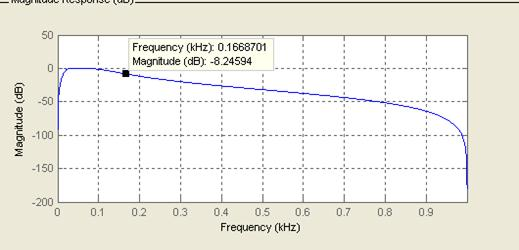Frequency Response for 2nd Order Butterworth with band f = [230 430] – high E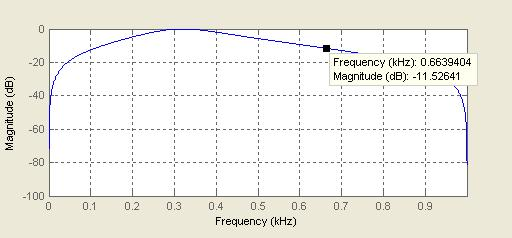Program/Hardware Design: Software Functionality

Initialization - void initialize(void)

First, set up Timer0 to run at 2kHz. Then set up Timer2 to fast PWM mode so it displays the waveform of the digitally filtered signal. Then, enable ADC and set the ADC prescaler to 128. Set up PORTA as input because we are using the ADC and the rest of the inputs are connected to switches used to select which string to tune. Initialize the program to be not in tune and motor not moving. Next, the program calls function KillEmAll(); to initialize other state variables. PORTB is set up as output to control the motor. The outputs from PORTB are connected to the motor driver chip. The unused logical inputs on the chip must be tied to ground or Vcc. PORTB.0 is set to zero because reset is not used. PORTB.1 controls the direction of which the motor spins. PORTB.2 and PORTB.3 control the step size of the motor. Since the torque should be maximized on this motor, the step size is set to 1/16 of a whole step. The sync function on the chip is not used, therefore PORTB.4 is set to zero. PORTB.5 is the clock of the chip. The chip will only work when there is a rising clock edge from the clock input. So PORTB.5 is toggled when the program wants the motor to move. The variable time1 is initialized to 1000 so the function ReighInBlood() can run at 2Hz. Finally, set up PORTC as output because the LCD is connected to it, set up PORTD.7 as the DAC output, initialize the LCD display and turn on the interrupts.

String Selection - void ReignInBlood(void) – running at 2Hz

This part of the software is the slowest process running on the MCU. The purpose of this piece of code is to let the user choose which string is being tuned. Due to the band-limiting filter at the input of the ADC, the higher strings tend to be attenuated. Therefore, two levels of control are in place in this function. First, the input signal to ADC can either come from the second amplifier or the first (the second amplifier is a further amplification of the output from the first). In this case, the program chooses between two ADC ports, ADC0 and ADC1, with ADC0 being the signal without extra amplification. Second, when doing the digital filtering, the signal can be filtered once or filtered by cascading two filters. It turns out that for the lowest two strings, ADC0 and cascading filters yield the best result; for the middle two strings, ADC0 with a single filter works the best; for the highest two strings, ADC1 with a single filter produce the best response. For each string, the filtering coefficients and the corresponding frequency boundaries will be set. The frequency boundaries (realFH and realFL) are set to within 1% of the real frequency (the choice of 1% will be discussed in the accuracy section) and are used to controlled the motor. Another set of boundaries (H and L) are set to within 5% of the real frequency. The reason being a half step corresponds to 2^(1/12) which is about 5%. For each string, a flag is set (start=1) to indicate that the tuning process can start.

Filtering & Motor Control - interrupt [TIM0_COMP] void timer0_compare(void) – running at 2kHz

Once the starting flag is set, the program enters into the code. First, if the guitar is not in tune, the motor has been started, and the motor still has steps left, the motor will move. The time variable merely keeps track of the time since the last calculation of frequency. Next, the ADC input will be read. The reason for subtracting 128 from the reading is because the filter uses signed numbers while the ADC reading ranges from 0 to 255 unsigned. After storing the ADCH in x0, another ADC reading starts. The next line outputs the filter result to DAC so it can be read on the oscilloscope. Then, depends which string it is, x0 is either filtered by a single filter or two filters cascaded together. Here we used the and modified the IIR2 Butterworth filter code written by Cornell University professor Bruce Land. The next few lines are used to keep track of previous outputs from the filter. These state variables are used in the zero-crossing scheme to improve accuracy. Finally, the next block of code will count the zero-crossings and determine the motor movement. First, if a crossing has occurred, the code will increment the cycle count. Once 10 crossings have occurred, the program will calculate the frequency. If the calculated frequency is within one half note of the desired frequency, the program will determine the motor movement. If the calculated frequency is 1% higher than the real frequency, the motor will move CCW; if the calculated frequency is 1% lower than the real frequency, the motor will move CW; if the calculated frequency is within 1% of the real frequency, the motor will stay put.

Frequency Display - void lcdcdcd(void) – running at 10Hz

This function merely displays the calculated the frequency if it's within 5% of the actual frequency of the string.

Motor, Motor Control

The motor that is used in this project is a 6-wire unipolar stepper motor. First we had to figure out what each wire did. This was done by measuring the resistance between different combinations of two wires. If two wires have infinite resistance between them, they are from two different coils; if two wires have half the resistance of some other combinations, it means one of the two wires is connected to the voltage source; if two wires have the whole resistance, they are the two ends of the same coil. Next, we needed to figure out the phase of the wires so we could connect the motor to the motor driver chip. So we had to connect both power wires to a voltage source. Then choose any of the 4 wires that are left and connect it to ground. Then ground the last three wires in sequence to see which one makes it turn CW, which one makes makes it turn CCW, and which one does nothing. Make a chart for all four wires, then figure out the sequence of wire combinations that makes the motor turn CW or CCW. Once the motor is figured out, we can move on to the motor driver. The driver chip has two sides. One side of the chip communicates with the MCU and the other side controls the motor. The motor controlling side requires at least 10V to function. This was a problem because the motor was only rated at 5V. We let 10V run through the motor, and we didn't burn the motor, so we assumed that it was safe. The example circuitry for the motor driver chip looks taunting at first. After close examination of the data sheet, however, we realized that we didn't need to implement everything the data sheet had suggested. This simplification saved us one pMOS transistor and dramatically reduced our workload. After scoping each output, and figuring out the phase difference between each output, we could connect the motor like so: the two ends on the same coil should have 180 degree phase difference while the ends on the same side of different coils should have 90 degree phase difference.

Below is the schematic for the motor driver circuit taken from the data sheet: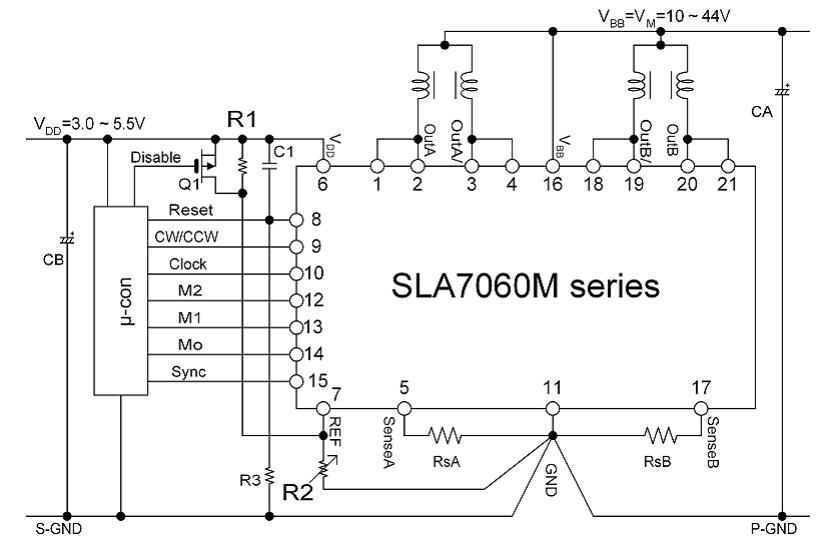Results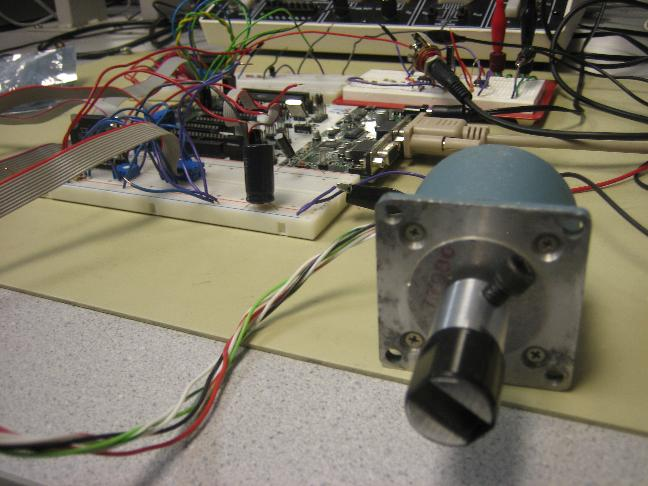SPEED OF EXECUTION & reliability

The tuner takes some time to tune a string, as the DSP waits for a reading in the range of half a note within the desired fundamental harmonic.  Thus, the tuning

process can be slow.  At times the result may be incorrect and it may take more than once time to tune a string correctly.  This situation occurs more frequently

when tuning the B string, as it is of an incorrect thickness.

Accuracy

We had a number of accuracy issues to deal with in this project. First of all, we have the accuracy issues on the DSP side: the quantization of the original guitar signal by the ADC and the attenuation of the signal by the digital filter. Then we have a motor which is inaccurate up to 3%. This becomes a huge issue for this project because each half note is only 5% apart and a human with perfect pitch can actually detect up to 0.1% or about 3 cents of inaccuracy. To counter all these obstacles, we let the motor move in very fine steps and smaller overall movements but more frequently. The string is tuned when the motor starts to turn back and forth around a certain point. This turned out to work well.

USABILITY

Our device is somewhat cumbersome, with many fragile wires and stray cables.  I would say that few people other then ourselves would be able to use this

device in its current state.

Conclusions

Results of our design versus our initial expectations

Through the course of our project our expectations were continually revised as we encountered the practical limitations and constraints that we had to work with. We envisioned initially, for instance, 6 motors simultaneously tuning all the strings; this initial vision was quickly abandoned upon realizing how bulky and inelegant it would be to clamp 6 motors to the head of a guitar. Also, accuracy of tuning did not appear to be a big issue initially, as we supposed the process of counting zero crossings to be fairly straightforward; we discovered through our design process, however, that allowing a small margin of error (~1%) in tuning the frequency was an unavoidable concession that we had to make. One thing we might do differently next time is to use a different filtering scheme (e.g. a set of three closely spaced bandpass filters to determine frequency by comparing the three filter responses, rather than calculating the frequency based on the output of a single bandpass filter) which might improve the accuracy of our frequency calculations.

Conformation of our design to the applicable standards

Our code easily conformed to the ANSI C and IEEE 32-bit floating point standards.

Intellectual property considerations

We did not reuse code or someone else’s design, although we read previous ECE476 guitar tuner project reports, and the method of counting zero crossings was suggested to us by Prof Bernard Hutchins. We did not reverse-engineer other designs and did not have to deal with patent/trademark issues. Sampling of the motor driver chip was also hassle-free and required no non-disclosures. We think our project is patentable; its usefulness is obvious, and it is somewhat surprising that there is not a product like this on the market already.

Ethical considerations

To the best of our knowledge, we did not violate any of the rules on the IEEE code of ethics.  Our motor drew a lethal 1 A current; however, we made sure that the circuit was closed and that we did not touch the motor circuit while it was on.  Therefore, the device is safe for general use, though as a product would require more stringent requirements.  In terms of crediting others for our work, we did make an effort to thank the persons who machined the motor driveshaft as well as Bruce Land, who gave us a lot of good ideas and criticism.  If we were to develop this as a real product, we would explore new ideas and methods and credit them as well.  We were honest in our reporting of accuracy and reliability, and did not attempt to hide our drawbacks.  Once we patent this idea, we wish to sell the design and accept full responsibility for any design flaws.  We will not attempt to misrepresent any data or testing results.  In requesting samples or free parts, we were not bribed or paid by any person or company to use their specific part.

Legal considerations

We did not encounter any legal restrictions in the course of our project.

Different Approaches, Failures, Difficulties:

1.      We initially tried to implement a state-machine based motor control scheme. The idea was to let the frequency calculations happen, and then decide what to do after the frequency settled on one value. This didn't work out well because the frequency calculations are not that reliable. We decided that the better approach would be to let the motor move more frequently but in smaller steps so that overall the motor moves towards the right direction.

2.      We thought we would need a gear train to turn the guitar because stepper motors are known to have weak torques. After consulting Professor Moon and Rick Schmidt from M&AE, we realized that a gear train would be way too expensive to implement for this project and we only needed a strong stepper motor which we got from Rick Schmidt.

Appendix: Parts list and cost details

 Part Part number Quantity used Cost (\$) Source STK500 board STK500 1 15 Rented Atmel mega32 mcu ATmega32 1 8 Rented White board N/A 2 12 Rented LCD LCD 1 8 Rented DIP socket N/A 1 0.50 Rented 1-pin jumper cable N/A 10 5 Rented Power supply N/A 2 5 Rented Op-amp LM358 2 0 Rented M-Audio Preamp Audio Buddy 1 0 Own Motor LM061-FD02 1 0 Scavenged Motor driver chip SLA7060M 1 0 Sampled Claw N/A 1 0 Outsourced Total Cost: \$53.50 Balanced Budget Yes

Appendix: Distribution of tasks among team members

We collaborated on all aspects of the project, though each of us did ‘specialize’ in different aspects. Alex was most familiar with the coding; Ayan was most familiar with the filter design process; Paul dealt mostly with building the hardware. All of us tested, debugged and refined our guitar tuner in lab together.

References

Data sheets

Mega32:

Motor driver:

Motor:

Vendor sites

Motor driver:

Code/designs borrowed from others

DSP code from Prof Bruce Land:

Background sites

Previous 476 guitar tuner projects:

Analog filter design:

DSP on mega32:

Appendix: Commented Software Code

//time base will be 10e-4

//stuff that needs to be added in:

//

//

//PORT hookups:

//Port A: ADC

//Port B: LED outputs

//Port D: pushbutton inputs

#asm

.equ __lcd_port=0x15

#endasm

#include <lcd.h> // LCD driver routines

#include <Mega32.h>

#include <stdio.h>

#define begin {

#define end   }

#define float2fix(a) ((int)((a)*256.0))

#define fix2float(a) ((float)(a)/256.0)

#define int2fix(a)   (((int)(a))<<8)

int  time, cycle, timerecorded, hc, start, L, H, th, step;

char inTune, motorS;

signed int x0, y0, y1, y2,y3,y4,y5,y6,y7,y8,y9,thre, thre1, TT;

signed int P1, P0;

char lcd_buffer;

float frequency, realF, realFH, realFL, af;

int time1, timelcd;

int bias;

void initialize(void);

int IIR2(int);

int OnePoleLow(int);

void KillEmAll(void);

void ReignInBlood(void);

//*******************************************************

#pragma regalloc-

int b1,a2,a3,a4,a5;   //filter constants

int b2,b3,b4,b5;

int xn, xn_1, xn_2, xn_3, xn_4,  ; //input state

int yn, yn_1, yn_2, yn_3, yn_4  ; //output state

int alpha, ym_1;

#pragma regalloc+

//*****************************************************

//*****************************************************

//timer 0 compare ISR

interrupt [TIM0_COMP] void timer0_compare(void)

begin

//function counters

if (time1 > 0) --time1;

if (timelcd > 0) --timelcd;

//digital filtering & motor control

if(start==1)

begin

//guitar not in tune, motor has been started and still has steps left

//then move the motor!

if (inTune==0 && motorS==1 && step>0)

begin

step--;

PORTB.5 = ~PORTB.5;

end

//incrementing time counter

time++;

//get ADC reading from ADCH, start another reading

x0=(int) (ADCH-128);

ADCSR.6=1;

//output to DAC to see the filtered waveform

OCR2 = (y0>>1) + 127;

//decide if one filter should be used or cascading two filters

if (hc==0)

begin

//single filter

y0=IIR2(x0);

end

else if (hc==1)

begin

//two filters cascaded

y0=IIR2(IIR2(x0));

end

//update state variables

y3=y2;

y2=y1;

y1=y0;

//counting zero crossings

if((y0>th)&& (y1 >=th) && (y2<=th) && (y3 <th))

begin

//update number of cycles for each crossing

cycle++;

//when 10 cycles are reached execute the following code

if (cycle==10)

begin

cycle=0;    //reset cycle count

frequency = 20000.0 /((float) time);    //calculating the frequency using 10 cycles time

time=0; //reset timer and begin measurement for next 10 periods

//when the frequency is within the range

if ((frequency < H) && (frequency >L))

begin

//when the frequency is higher, motor moves to make it flatter

if (frequency > realFH)

begin

PORTB.1 = 0; //direction

inTune = 0; //not in tune

motorS = 1; //start the motor

step = 300; //set the number steps

end

//when the frequency is lower, motor moves to make it sharper

else if (frequency < realFL)

begin

PORTB.1 = 1; //direction

inTune = 0; //not in tune

motorS = 1; //start the motor

step = 300; //set the number steps

end

//when the freuency is in tune, motor doesn't move

else

begin

inTune = 1; //in tune

motorS = 0; //stop the motor

end

end

end

end

end

end

//========================================================

//second order IIR -- "Direct Form II Transposed"

//  y(n) = b(1)*x(n) + b(2)*x(n-1) +  b(3)*x(n-2)

//                   - a(2)*y(n-1) -  a(3)*y(n-2)

//assumes a(1)=1

// a's and b's need to be global, in RAM and set to fixed point values

// also input and output history values

//example:

//      #pragma regalloc-

//      int b1,b2,b3,a2,a3, xn_1, xn_2, yn_1, yn_2 ;

//      #pragma regalloc+

//      b1=0x0010;

//      a1=float2fix(-(value from matlab))

//

//========================================================

///////////////////////////////////////////////////////

int IIR2(int xx)

// xx is the current input signal sample

// returns the current filtered output sample

begin

#asm

.macro mult_acc         ;r31:r30:r24 += r23:r22 * r21:r20

muls r23, r21       ; (signed)ah * (signed)bh

add r31, r0

mul r22, r20        ; al * bl

add r24, r0

adc r30, r1

adc r31, r27

mulsu   r23, r20        ; (signed)ah * bl

add r30, r0

adc r31, r1

mulsu   r21, r22    ; (signed)bh * al

add r30, r0

adc r31, r1

.endm

push r20    ;save parameter regs

push r21

clr r27             ;permanent zero

clr r24             ;clear 24 bit result reg; msb to lsb => r31:r30:r24

clr r30

clr r31

lds  R22, _b1       ;load b1 from RAM

lds  R23, _b1+1

ld   R20, Y         ;load input parameter xx from stack

ldd  R21, Y+1

mult_acc            ; b1*xx

lds  R22, _b2       ;load b2 from RAM

lds  R23, _b2+1

lds  R20, _xn_1     ;load x(n-1) from  RAM

lds  R21, _xn_1+1

mult_acc            ; b2*x(n-1)

lds  R22, _b3       ;load b3 from RAM

lds  R23, _b3+1

lds  R20, _xn_2     ;load x(n-2) from  RAM

lds  R21, _xn_2+1

mult_acc            ; b3*x(n-2)

lds  R22, _a2       ;load -a2 from RAM

lds  R23, _a2+1

lds  R20, _yn_1     ;load y(n-1) from  RAM

lds  R21, _yn_1+1

mult_acc            ; -a2*y(n-1)

lds  R22, _a3       ;load -a3 from RAM

lds  R23, _a3+1

lds  R20, _yn_2     ;load y(n-2) from  RAM

lds  R21, _yn_2+1

mult_acc            ; -a3*y(n-2)

lds  R20, _xn_1     ;load x(n-1) from  RAM

lds  R21, _xn_1+1

sts  _xn_2, r20      ;store x(n-2) to  RAM

sts  _xn_2+1, R21

ld   R20, Y         ;load input parameter xx from stack

ldd  R21, Y+1

sts  _xn_1, r20     ;store x(n-1) to  RAM

sts  _xn_1+1, R21

lds  R20, _yn_1     ;load y(n-1) from  RAM

lds  R21, _yn_1+1

sts  _yn_2, R20     ;store y(n-2) to  RAM

sts  _yn_2+1, R21

sts  _yn_1, r30     ;store new output as y(n-1) to  RAM

sts  _yn_1+1, r31

pop r21             ;restore parameter regs

pop r20

#endasm

end

#pragma warn+

//initialization

void initialize(void)

begin

TIMSK = 2;              //timer0 compare match interrupt

OCR0 = 125;             //count up to 25

TCCR0 = 0b00001011;     //prescale by 64

TCCR2 = 0b01111001;     //fast PWM to display filtered signal

ADCSR = 0b11000111;     //init the A to D converter

//set up PORTA as input

//ADC and switches

//start all off

DDRA = 0x00;                                 //input pushbuttons

PORTA = 0xff;

//initialize state variables

//not in tune

//not starting motor

inTune = 0;

motorS = 0;

//more initialization

KillEmAll();

//set up PORTB as output to the motor driver chip

DDRB = 0xff;

PORTB.0 = 0; //reset

PORTB.1 = 0; //direction

PORTB.2 = 0; //M2

PORTB.3 = 0; //M1

PORTB.4 = 0; //sync

PORTB.5 = 0; //clock

//initilize time counter for ReignInBlood

time1 = 1000;

//don't start the filtering yet

start = 0;

//set up PORTD.7 as the DAC output

DDRD.7 = 1;

//set up the LCD display

DDRC = 0xff;

lcd_init(16);

lcd_clear();

//start the interrupts

#asm

sei

#endasm

end

//reset state variables

void KillEmAll(void)

begin

y1=y2=y3=y0=0;

time=0;

cycle=0;

inTune = 0;

motorS = 0;

end

//selecting which string

void ReignInBlood(void)

begin

//reset the counter

time1=1000;

//choosing which string

//set the ADMUX for each string

//set the filtering coefficients for each string

//reset the state variables by calling KillEmAll

//set the 5% boundaries

//set the real frequency for each string

//set the threshold for each string

//start the digital filtering

//determine if using one filter or cascading two filters

if (!PINA.2)

begin

ADMUX = 0b01100000;

start=0;

b1 = float2fix(0.1367);

b2 = float2fix(0);

b3 = float2fix(-0.1367);

a2 = float2fix(1.7059);

a3 = float2fix(-0.7265);

KillEmAll();

L=77;

H=88;

realF = 82.407;

th=0;

start=1;

hc =1;

end

if (!PINA.3)

begin

ADMUX = 0b01100000;

start=0;

b1 = float2fix(0.2043);

b2 = float2fix(0);

b3 = float2fix(-0.2043);

a2 = float2fix(1.5626);

a3 = float2fix(-0.5914);

KillEmAll();

L=103;

H=117;

realF = 110;

th=0;

start=1;

hc =1;

end

if (!PINA.4)

begin

ADMUX = 0b01100000;

start=0;

b1 = float2fix(0.2836);

b2 = float2fix(0);

b3 = float2fix(-0.2836);

a2 = float2fix(1.3730);

a3 = float2fix(-0.4327);

KillEmAll();

L=138;

H=156;

realF = 146.83;

th=2;

start=1;

hc =0;

end

if (!PINA.5)

begin

ADMUX = 0b01100000;

start=0;

b1 = float2fix(0.1367);

b2 = float2fix(0);

b3 = float2fix(-0.1367);

a2 = float2fix(1.3979);

a3 = float2fix(-0.7265);

KillEmAll();

L=185;

H=208;

realF = 196;

th=0;

start=1;

hc =0;

end

if (!PINA.6)

begin

ADMUX = 0b01100001;

start=0;

b1 = float2fix(0.1367);

b2 = float2fix(0);

b3 = float2fix(-0.1367);

a2 = float2fix(1.2361);

a3 = float2fix(-0.7265);

KillEmAll();

L=233;

H=262;

realF = 246.9;

th=0;

start=1;

hc =0;

end

if (!PINA.7)

begin

ADMUX = 0b01100001;

start=0;

b1 = float2fix(0.1367);

b2 = float2fix(0);

b3 = float2fix(-0.1367);

a2 = float2fix(0.8898);

a3 = float2fix(-0.7265);

KillEmAll();

L=311;

H=349;

realF = 329.6;

th=2;

start=1;

hc =0;

end

//set the 1% boundaries for the frequency

realFH = realF * 1.01;

realFL = realF * 0.99;

end

//display the filtered frequency

void lcdcdcd(void)

begin

//runs at 10hz

timelcd=200;

//if frequency is within 5%, display it

if (!((frequency>H)||(frequency<L)))

begin

lcd_clear();

lcd_gotoxy(0,0);

sprintf(lcd_buffer,"F: %f", frequency);

lcd_puts(lcd_buffer);

end

end

void main(void)

begin

//initialization

initialize();

//call the functions

while (1)

begin

if(timelcd==0)  lcdcdcd();

if(time1==0) ReignInBlood();

end

end